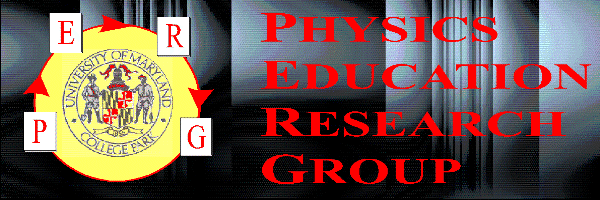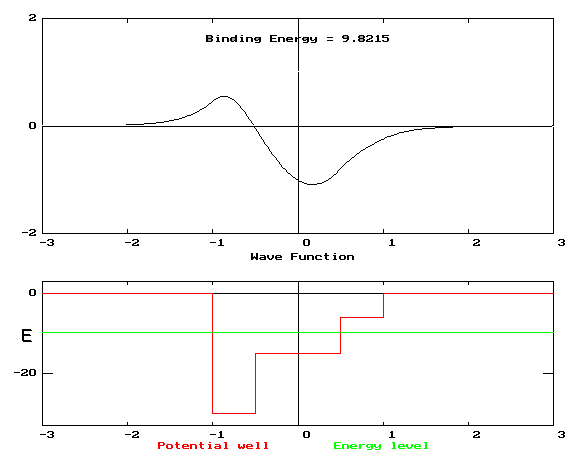# Student Difficulties with Energy in Quantum Mechanics

and

## Pratibha Jolly

### University of DelhiSupported in part by NSF grant DUE-9455561.

Presented 6 January, 1997 at the Phoenix meeting of the AAPT. Posted on the Web on 22 January, 1997.

## Abstract

The Physics Education Research Group at University of Maryland has been studying student learning of quantum mechanics. Our previously reported research shows that student difficulties exist with classical concepts that are prerequisite for learning quantum mechanics. In this talk, we report detailed studies of student difficulties in quantum mechanics arising from confusions with the classical concept of energy. Students are confused on some detailed issues concerning energy and energy diagrams such as the possible value of total classical energy, the meaning of discontinuous potential energy diagrams, and the quantization of energy levels in the quantum case. We have also found new induced confusions on classical issues that are created by students misinterpreting quantum concepts. Proposals for instruction to deal with these issues will be discussed.

## Presentation

A number of factors make it both desirable and possible to teach quantum mechanics to more students and at an earlier stage.

• New technology dependent on the quantum nature of matter.
• Increased computer availability that permits improved models with less abstract math.
• A new prospective audience of research engineers (electrical and materials) and molecular chemists and biologists.

If we are to reach this new audience with these new tools, we must take into account the nature of the audience. (speculation)

• A tendency to more concrete and less mathematical inclinations than the typical physicist
• A lack of willingness to "postpone gratification" till the 3rd semester
• A weaker background in formal physics than the typical incoming physics grad student.
To reach these students we need to understand

• their initial state
• how they respond to instruction

The UMd PERG has begun preliminary investigations of student response to instruction in QM.

Context: the third semester of a 3 semester calculus-based introductory physics course for engineers

• 3 hrs of lecture
• 2 hrs of lab
• 1 hr of tutorial (UW style)

Instruction: approximately 1/3 of the course's lectures (5 weeks) were de-voted to QM. In addition, we developed 3 tutorials.

building potential wells
MBL tutorial with cart moving along a track. Force probe + sonic ranger give F vs. x plot. Students build PE graph and con-sider classical probability.
understanding eigenvalues
Computer simulation (MUPPET). Students solve Schr. eqn. at various energies and find only a few give allowable wave functions.
the shape of the wave function
Computer simulation (MUPPET). Students explore relation between local KE and w. fn. curvature.

Research was based upon

• pre-tests
• homework
• interviews with 6 students (all were A students)
• a final exam question

Most students can relate the energy diagram with local velocity and many can make the connections to classical probability density.

Students scored much better than ex-pected on a final exam problem (multiple-choice multiple-response) that asked them to choose classical and quantum probabilities for a particle moving in a complex well.

### Exam Problem

In the figure below is shown a plot of a one-dimensional potential energy function U[x] (lower plot) and the wavefunction (upper plot) of an eigenstate for an electron in the influence of that potential. The energy, E, associated with that state is also shown on the energy plot. Distances are measured in nanometers and energies in eV.1.1 If the particle were moving classically (i.e., its motion were described by Newton's laws) in the potential U[x], and it had an energy E, which of the following statements would be true? List all that apply.

• (a) It would move the fastest when it was in region II.
• (b) It would move the fastest when it was in region III.
• (c) If we took a photograph of the particle at a random time, we would never find it in re-gion IV.
• (d) If we took a photograph of the particle at a random time, we would be most likely to find it in region II.
• (e) If we took a photograph of the particle at a random time, we would be most likely to find it in region III.

1.2 If the particle were moving quantum mechanically (i.e., its motion were described by the Schrödinger equation) in the potential U[x], and it had an energy E, which of the fol-lowing statements would be true? List all that apply.

• (a) If we measured the position of the particle at a random time, we would never find it in region I.
• (b) If we measured the position of the particle at a random time, we would never find it in region IV.
• (c) If we measured the position of the particle at a random time, we would most likely find it in region II.
• (d) If we measured the position of the particle at a random time, we would most likely find it in region III.
• (e) The state shown represents the lowest energy state that can be found in this well.

Although students scored well on the multiple-choice multiple-response exam question (81%), pre-tests, homeworks, and interviews indicated that even the top students had a number of serious difficulties.

• concept of probability density
• understanding of energy issues associated with potential wells and quantum states

### Interview protocol:

Students were (multiple region) formula specifying a potential well similar to the one on the final. They had to

• draw the PE diagram
• interpret the diagram ("tell its story")
• discuss the set of allowable energies, classically and quantum mechanically
• discuss the motion of the particle classically
• discuss the probability of finding the particle in different regions classically and quantum mechanically

### Observations:

The group of 6 students averaged 92% on the MCMR question. There was much that was reasonable in the interviews, but...

1. 2/6 students had serious difficulty with the discontinuously defined function.
2. Only one correctly stated the classically allowed energies (E> -30 eV).
3. Some thought that the quantum energies could only have negative energies and only 2/6 stated that the energy should be discrete.

Some students had difficulty with the discontinuously defined function.

S: [The vertical lines?] Well, I was really connecting the different energy states. Because I wanted this guy to be continuous....I think they belong there. Yeah...the transition. The transitions from one state to another.

S': The...umm...the borders of the well...at those...classically, an electron can't be there.

S'': Transitions between different states, I believe.

Some students had difficulty interpreting negative energies.

I: For a classical system...are there any values of the total energy that the particle in the system could not have?

S: In a classical system, we can not have negative energies, so you can have any-thing greater than or equal to zero but not anything less. Classically.

S': ...total energy is going to be ... has to be positive [later accepts request to draw a negative energy and interprets motion and prob-ability approximately correctly]

S'':...the whole thing with negative energy was funny to me.

Some students had difficulty under-standing what energies were allowed in a potential energy diagram, both in classical and quantum mechanics. When asked if there were limitations on the allowed energies in QM, only one student gave the correct answer.

S': No, there is no limitation. The limitation again has to be the floor. See you can’t go below the floor. Yeah, which would mean you can’t go below -30. So the same limitation as in the classical case.

S'': It can have ..… negative or positive ….. well it can, any where between zero and negative thirty. But it couldn’t have a positive value.

### Conclusions:

• It is possible to teach some Schrödinger level QM to sophomore engineers with good success as measured by reasonably difficult final exam questions.

• Success on such questions does not mean that students have understood the fundamental concepts or created a coherent picture.

• Difficulties can be caused by classical concepts (such as energy or probabil-ity) which are often assumed but which may be poorly understood.

Stay tuned!